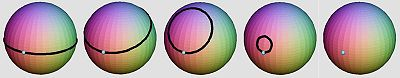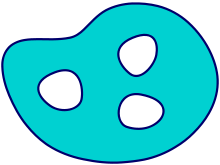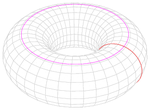# Simply connected space

﻿
Simply connected space

In topology, a topological space is called simply connected (or 1-connected) if it is path-connected and every path between two points can be continuously transformed, staying within the space, into any other path while preserving the two endpoints in question (see below for an informal discussion).

If a space is not simply connected, it is convenient to measure the extent to which it fails to be simply connected; this is done by the fundamental group. Intuitively, the fundamental group measures how the holes behave on a space; if there are no holes, the fundamental group is trivial — equivalently, the space is simply connected.

## Informal discussion

Informally, a thick object in our space is simply connected if it consists of one piece and does not have any "holes" that pass all the way through it. For example, neither a doughnut nor a coffee cup (with handle) is simply connected, but a hollow rubber ball is simply connected. In two dimensions, a circle is not simply connected, but a disk and a line are. Spaces that are connected but not simply connected are called non–simply connected or, in a somewhat old-fashioned term, multiply connected.A sphere is simply connected because every loop can be contracted (on the surface) to a point.

To illustrate the notion of simple connectedness, suppose we are considering an object in three dimensions; for example, an object in the shape of a box, a doughnut, or a corkscrew. Think of the object as a strangely shaped aquarium full of water, with rigid sides. Now think of a diver who takes a long piece of string and trails it through the water inside the aquarium, in whatever way he pleases, and then joins the two ends of the string to form a closed loop. Now the loop begins to contract on itself, getting smaller and smaller. (Assume that the loop magically knows the best way to contract, and won't get snagged on jagged edges if it can possibly avoid them.) If the loop can always shrink all the way to a point, then the aquarium's interior is simply connected. If sometimes the loop gets caught — for example, around the central hole in the doughnut — then the object is not simply connected.

Notice that the definition only rules out "handle-shaped" holes. A sphere (or, equivalently, a rubber ball with a hollow center) is simply connected, because any loop on the surface of a sphere can contract to a point, even though it has a "hole" in the hollow center. The stronger condition, that the object have no holes of any dimension, is called contractibility.

## Formal definition and equivalent formulationsThis set is not simply connected because it has three holes.

A topological space X is called simply connected if it is path-connected and any continuous map f : S1X (where S1 denotes the unit circle in Euclidean 2-space) can be contracted to a point in the following sense: there exists a continuous map F : D2X (where D2 denotes the unit disk in Euclidean 2-space) such that F restricted to S1 is f.

An equivalent formulation is this: X is simply connected if and only if it is path-connected, and whenever p : [0,1] → X and q : [0,1] → X are two paths (i.e.: continuous maps) with the same start and endpoint (p(0) = q(0) and p(1) = q(1)), then p and q are homotopic relative {0,1}. Intuitively, this means that p can be "continuously deformed" to get q while keeping the endpoints fixed. Hence the term simply connected: for any two given points in X, there is one and "essentially" only one path connecting them.

A third way to express the same: X is simply connected if and only if X is path-connected and the fundamental group of X at each of its points is trivial, i.e. consists only of the identity element.

Yet another formulation is often used in complex analysis: an open subset X of C is simply connected if and only if both X and its complement in the Riemann sphere are connected.

The set of complex numbers with imaginary part strictly greater than zero and less than one, furnishes a nice example of an unbounded, connected, open subset of the plane whose complement is not connected. It is nevertheless simply connected. It might also be worth pointing out that a relaxation of the requirement that X be connected, leads to an interesting exploration of open subsets of the plane with connected extended complement. For example, a (not necessarily connected) open set has connected extended complement exactly when each of its connected components are simply connected.

## ExamplesA torus is not simply connected. Neither of the colored loops can be contracted to a point without leaving the surface.
• The Euclidean plane R2 is simply connected, but R2 minus the origin (0,0) is not. If n > 2, then both Rn and Rn minus the origin are simply connected.
• Analogously: the n-dimensional sphere Sn is simply connected if and only if n ≥ 2.
• Every convex subset of Rn is simply connected.
• A torus, the (elliptic) cylinder, the Möbius strip, the projective plane and the Klein bottle are not simply connected.
• Every topological vector space is simply connected; this includes Banach spaces and Hilbert spaces.
• The special orthogonal group SO(n,R) is not simply connected for n ≥ 2; the special unitary group SU(n) is simply connected.
• The long line L is simply connected, but its compactification, the extended long line L* is not (since it is not even path connected).
• Similarly, the one-point compactification of R is not simply connected (even though R is simply connected).

## Properties

A surface (two-dimensional topological manifold) is simply connected if and only if it is connected and its genus is 0. Intuitively, the genus is the number of "handles" of the surface.

If a space X is not simply connected, one can often rectify this defect by using its universal cover, a simply connected space which maps to X in a particularly nice way.

If X and Y are homotopy equivalent and X is simply connected, then so is Y.

Note that the image of a simply connected set under a continuous function need not to be simply connected. Take for example the complex plane under the exponential map, the image is C - {0}, which clearly is not simply connected.

The notion of simple connectedness is important in complex analysis because of the following facts:

The notion of simply connectedness is also a crucial condition in the Poincaré lemma.

Wikimedia Foundation. 2010.

### Look at other dictionaries:

• Locally simply connected space — In mathematics, a locally simply connected space is a topological space that admits a basis of simply connected sets. Every locally simply connected space is also locally path connected and locally connected.The circle is an example of a locally… …   Wikipedia

• Connected space — For other uses, see Connection (disambiguation). Connected and disconnected subspaces of R² The green space A at top is simply connected whereas the blue space B below is not connected …   Wikipedia

• Simply connected at infinity — In topology, a branch of mathematics, a topological space X is said to be simply connected at infinity if for all compact subsets C of X , there is a compact set D in X containing C so that the induced map: pi 1(X D) o pi 1(X C),is trivial.… …   Wikipedia

• Locally connected space — In this topological space, V is a neighbourhood of p and it contains a connected neighbourhood (the dark green disk) that contains p. In topology and other branches of mathematics, a topological space X is locally connected if every point admits… …   Wikipedia

• N-connected space — n connected redirects here; for the concept in graph theory see Connectivity (graph theory). In the mathematical branch of topology, a topological space X is said to be n connected if and only if it is path connected and its first n homotopy… …   Wikipedia

• Semi-locally simply connected — In mathematics, in particular topology, a topological space X is called semi locally simply connected if every point x in X has a neighborhood U such that the homomorphism from the fundamental group of U to the fundamental group of X , induced by …   Wikipedia

• Timelike simply connected — Suppose a Lorentzian manifold contains a closed timelike curve (CTC). No CTC can be continuously deformed as a CTC (is timelike homotopic) to a point, as that point would not be causally well behaved. Therefore, any Lorentzian manifold containing …   Wikipedia

• Space Lords — is an arcade game released by Atari Games in 1992. It was a first person perspective space flight combat simulator. The gameplay allowed a player to fly in any direction he chose. It is notable in that it was, in fact, a multi player form of an… …   Wikipedia

• Connected sum — In mathematics, specifically in topology, the operation of connected sum is a geometric modification on manifolds. Its effect is to join two given manifolds together near a chosen point on each. This construction plays a key role in the… …   Wikipedia

• Space Shuttle Solid Rocket Booster — The Space Shuttle Solid Rocket Boosters (SRBs) are the pair of large solid rockets used by the Space Shuttle during the first two minutes of powered flight. They are located on either side of the orange external propellant tank. Each SRB produces …   Wikipedia